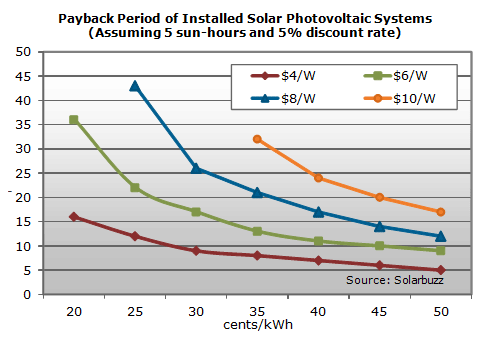# The Costs and Payback Period of Solar Panels

by

Solar power payback is the time it takes for the savings from using solar energy to pay for the original system. Determining this period requires comparing the price of grid drawn electricity per kilowatt-hour (kWh) to the cost of solar electricity per kWh. There are many factors affecting payback period, and each system’s set of factors are unique. Here are a few:

• system type generally, the bigger the system, the lower the cost per kWh
• cost of financing interest on loans
• location an area with more solar radiation will provide more electricity, and thus will have a faster solar energy payback time

Even though producing solar power is more expensive than drawing from the grid, the above factors can be altered to lower the cost per kWh through:

• subsidies or rebates on PV systems from utilities or government energy agencies
• preferable financing from banks, such as low interest loans specifically for PV systems
• tax incentives such as sales tax exemption on PV systems, state personal income tax credits or property tax exemptions

To calculate the cost per watt (\$/W) of your PV system, divide the cost of the system by the wattage. For example, a system producing 2200 kW per year that cost \$13,000 would have have a cost of \$6.2/W.From the graph, you can estimate the payback period of your PV system. If your grid-drawn electricity rate is US\$0.35 per kW hour, and your PV system cost is US\$6 per watt, then your system would pay for itself in about 13 years.